Train DQN Agent for Lane Keeping Assist

This example shows how to train a deep Q-learning network (DQN) agent for lane keeping assist (LKA) in Simulink®. For more information on DQN agents, see Deep Q-Network Agents.

The reinforcement learning environment for this example is a simple bicycle model for ego vehicle dynamics. The training goal is to keep the ego vehicle traveling along the centerline of the lanes by adjusting the front steering angle. This example uses the same vehicle model as in Lane Keeping Assist System Using Model Predictive Control (Model Predictive Control Toolbox). The ego car dynamics are specified by the following parameters.

m = 1575;   % total vehicle mass (kg)
Iz = 2875;  % yaw moment of inertia (mNs^2)
lf = 1.2;   % longitudinal distance from center of gravity to front tires (m)
lr = 1.6;   % longitudinal distance from center of gravity to rear tires (m)
Cf = 19000; % cornering stiffness of front tires (N/rad)
Cr = 33000; % cornering stiffness of rear tires (N/rad)
Vx = 15;    % longitudinal velocity (m/s)

Define the sample time Ts and simulation duration T in seconds.

Ts = 0.1;
T = 15;

The output of the LKA system is the front steering angle of the ego car. To simulate the physical limitations of the ego car, constrain the steering angle to the range [-0.5,0.5] rad.

u_min = -0.5;
u_max = 0.5;

The curvature of the road is defined by a constant 0.001 (${m}^{-1}$). The initial value for the lateral deviation is 0.2 m and the initial value for the relative yaw angle is –0.1 rad.

rho = 0.001;
e1_initial = 0.2;
e2_initial = -0.1;

Open the model.

mdl = 'rlLKAMdl';
open_system(mdl);
agentblk = [mdl '/RL Agent'];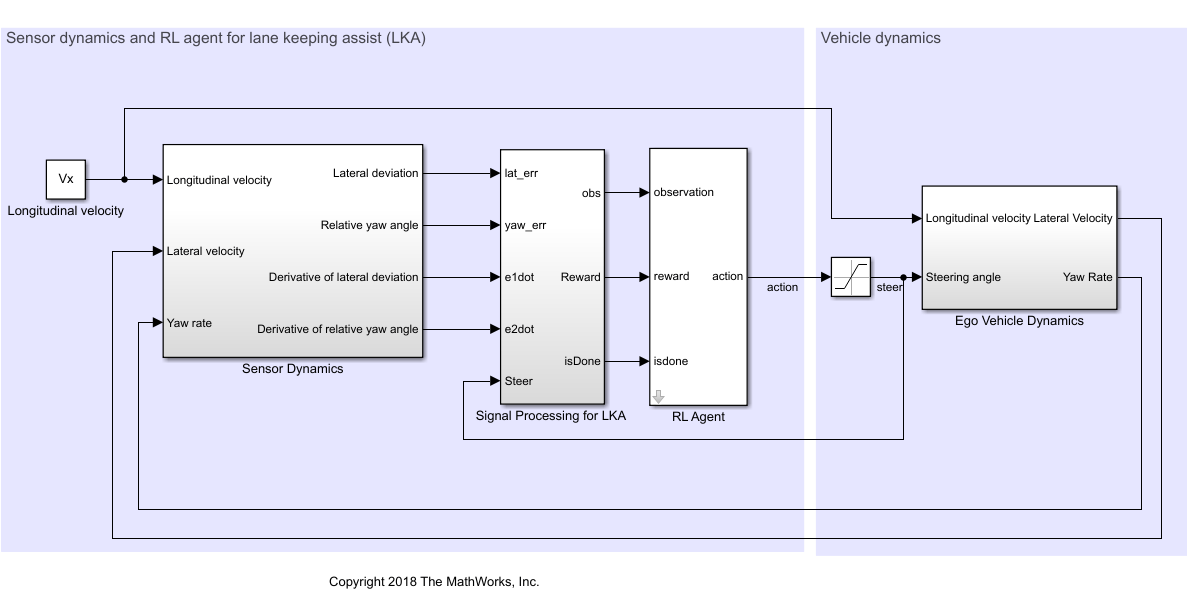For this model:

• The steering-angle action signal from the agent to the environment is from –15 degrees to 15 degrees.

• The observations from the environment are the lateral deviation ${\mathit{e}}_{1}$, the relative yaw angle ${\mathit{e}}_{2}$, their derivatives ${\underset{}{\overset{˙}{e}}}_{1}$ and ${\underset{}{\overset{˙}{e}}}_{2}$, and their integrals $\int {\mathit{e}}_{1}$ and $\int {\mathit{e}}_{2}$.

• The simulation is terminated when the lateral deviation $|{e}_{1}|>1.$

• The reward ${\mathit{r}}_{\mathit{t}}$, provided at every time step $\mathit{t}$, is

${r}_{t}=-\left(10{e}_{1}^{2}+5{e}_{2}^{2}+2{u}^{2}+5{\underset{}{\overset{˙}{e}}}_{1}^{2}+5{\underset{}{\overset{˙}{e}}}_{2}^{2}\right)$

where $\mathit{u}$ is the control input from the previous time step $\mathit{t}-1$.

Create Environment Interface

Create a reinforcement learning environment interface for the ego vehicle. To do so, first create the observation and action specifications.

observationInfo = rlNumericSpec([6 1],'LowerLimit',-inf*ones(6,1),'UpperLimit',inf*ones(6,1));
observationInfo.Name = 'observations';
observationInfo.Description = 'information on lateral deviation and relative yaw angle';
actionInfo = rlFiniteSetSpec((-15:15)*pi/180);
actionInfo.Name = 'steering';

Then, create the environment interface.

The interface has a discrete action space where the agent can apply one of 31 possible steering angles from –15 degrees to 15 degrees. The observation is the six-dimensional vector containing lateral deviation, relative yaw angle, as well as their derivatives and integrals with respect to time.

To define the initial condition for lateral deviation and relative yaw angle, specify an environment reset function using an anonymous function handle. This reset function randomizes the initial values for the lateral deviation and relative yaw angle.

env.ResetFcn = @(in)localResetFcn(in);

Fix the random generator seed for reproducibility.

rng(0)

Create DQN agent

A DQN agent approximates the long-term reward, given observations and actions, using a value function critic representation.

DQN agents can use multi-output Q-value critic approximators, which are generally more efficient. A multi-output approximator has observations as inputs and state-action values as outputs. Each output element represents the expected cumulative long-term reward for taking the corresponding discrete action from the state indicated by the observation inputs.

To create the critic, first create a deep neural network with one input (the six-dimensional observed state) and one output vector with 31 elements (evenly spaced steering angles from -15 to 15 degrees). For more information on creating a deep neural network value function representation, see Create Policy and Value Function Representations.

nI = observationInfo.Dimension(1);  % number of inputs (6)
nL = 24;                            % number of neurons
nO = numel(actionInfo.Elements);    % number of outputs (31)

dnn = [
featureInputLayer(nI,'Normalization','none','Name','state')
fullyConnectedLayer(nL,'Name','fc1')
reluLayer('Name','relu1')
fullyConnectedLayer(nL,'Name','fc2')
reluLayer('Name','relu2')
fullyConnectedLayer(nO,'Name','fc3')];

View the network configuration.

figure
plot(layerGraph(dnn))Specify options for the critic representation using rlRepresentationOptions.

Create the critic representation using the specified deep neural network and options. You must also specify the action and observation information for the critic, which you obtain from the environment interface. For more information, see rlQValueRepresentation.

critic = rlQValueRepresentation(dnn,observationInfo,actionInfo,...
'Observation',{'state'},criticOptions);

To create the DQN agent, first specify the DQN agent options using rlDQNAgentOptions.

agentOptions = rlDQNAgentOptions(...
'SampleTime',Ts,...
'UseDoubleDQN',true,...
'TargetSmoothFactor',1e-3,...
'DiscountFactor',0.99,...
'ExperienceBufferLength',1e6,...
'MiniBatchSize',64);

Then, create the DQN agent using the specified critic representation and agent options. For more information, see rlDQNAgent.

agent = rlDQNAgent(critic,agentOptions);

Train Agent

To train the agent, first specify the training options. For this example, use the following options:

• Run each training episode for at most 5000 episodes, with each episode lasting at most ceil(T/Ts) time steps.

• Display the training progress in the Episode Manager dialog box (set the Plots option to training-progress) and disable the command line display (set the Verbose option to false).

• Stop training when the episode reward reaches –1.

• Save a copy of the agent for each episode where the cumulative reward is greater than –2.5.

maxepisodes = 5000;
maxsteps = ceil(T/Ts);
trainingOpts = rlTrainingOptions(...
'MaxEpisodes',maxepisodes,...
'MaxStepsPerEpisode',maxsteps,...
'Verbose',false,...
'Plots','training-progress',...
'StopTrainingCriteria','EpisodeReward',...
'StopTrainingValue',-1,...
'SaveAgentCriteria','EpisodeReward',...
'SaveAgentValue',-2.5);

Train the agent using the train function. Training is a computationally intensive process that takes several hours to complete. To save time while running this example, load a pretrained agent by setting doTraining to false. To train the agent yourself, set doTraining to true.

doTraining = false;

if doTraining
% Train the agent.
trainingStats = train(agent,env,trainingOpts);
else
% Load the pretrained agent for the example.
end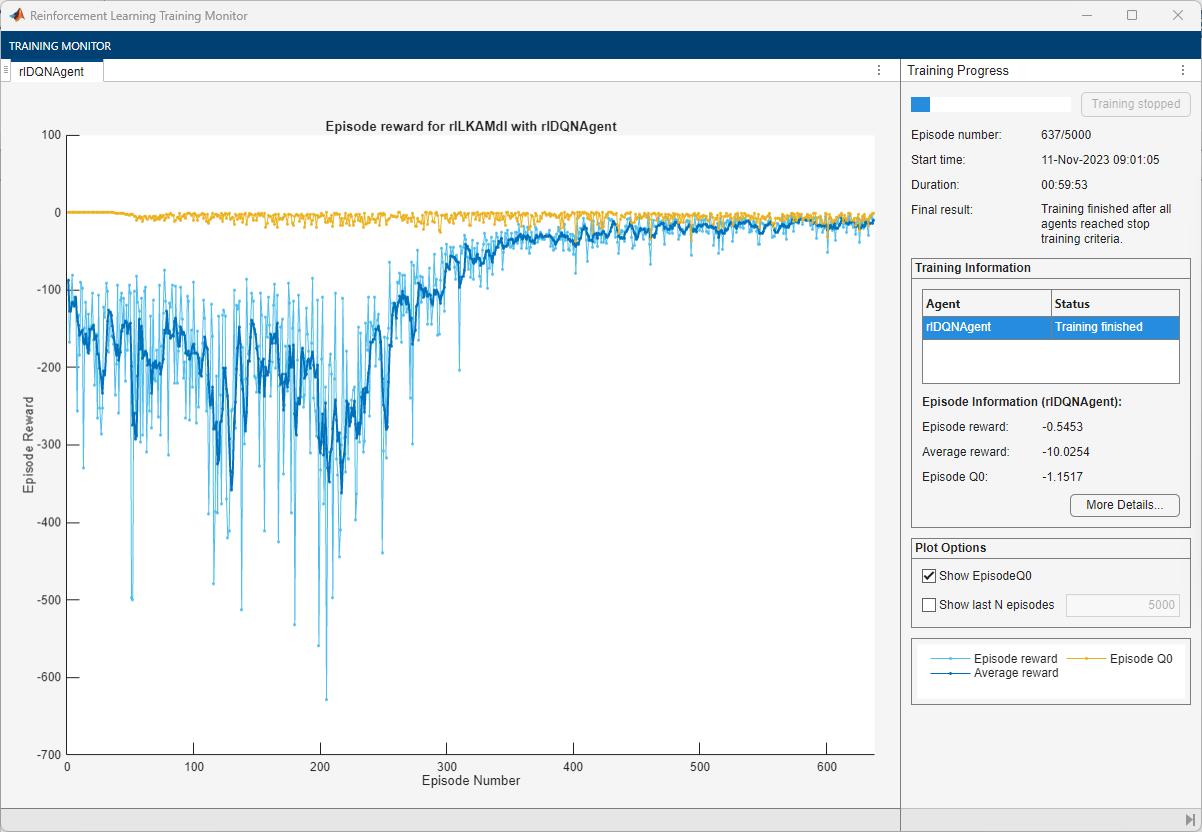Simulate DQN Agent

To validate the performance of the trained agent, uncomment the following two lines and simulate the agent within the environment. For more information on agent simulation, see rlSimulationOptions and sim.

% simOptions = rlSimulationOptions('MaxSteps',maxsteps);
% experience = sim(env,agent,simOptions);

To demonstrate the trained agent on deterministic initial conditions, simulate the model in Simulink.

e1_initial = -0.4;
e2_initial = 0.2;
sim(mdl)

As the plots show, the lateral error (top plot) and relative yaw angle (middle plot) are both driven close to zero. The vehicle starts from off the centerline (–0.4 m) and with a nonzero yaw angle error (0.2 rad). The lane keeping assist makes the ego car travel along the centerline after about 2.5 seconds. The steering angle (bottom plot) shows that the controller reaches steady state after about 2 seconds.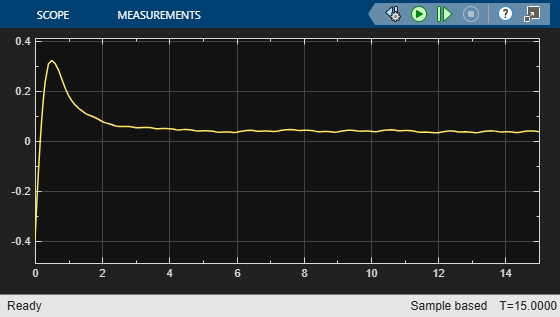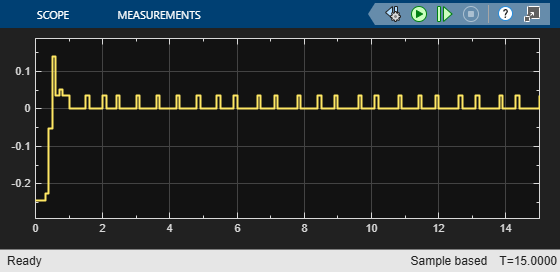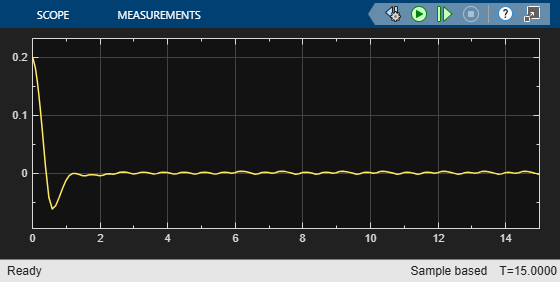if ~doTraining
%bdclose(mdl)
end

Reset Function

function in = localResetFcn(in)
% reset
in = setVariable(in,'e1_initial', 0.5*(-1+2*rand)); % random value for lateral deviation
in = setVariable(in,'e2_initial', 0.1*(-1+2*rand)); % random value for relative yaw angle
end